## Main

The asymmetry between matter and antimatter is related to the violation of the CP symmetry (CPV), where C and P are the charge-conjugation and parity operators. CP violation is accommodated in the standard model (SM) of particle physics by the Cabibbo–Kobayashi–Maskawa (CKM) mechanism that describes the transitions between up- and down-type quarks1,2, in which quark decays proceed by the emission of a virtual W boson and where the phases of the couplings change sign between quarks and antiquarks. However, the amount of CPV predicted by the CKM mechanism is not sufficient to explain our matter-dominated Universe3,4 and other sources of CPV are expected to exist. The initial discovery of CPV was in neutral K meson decays5, and more recently it has been observed in B0 (refs 6,7), B+(refs 8,9,10,11), and Bs0 (ref. 12) meson decays, but it has never been observed in the decays of any baryon. Decays of the Λb0 (bud) baryon to final states consisting of hadrons with no charm quarks are predicted to have non-negligible CP asymmetries in the SM, as large as 20% for certain three-body decay modes13. It is important to measure the size and nature of these CP asymmetries in as many decay modes as possible, to determine whether they are consistent with the CKM mechanism or, if not, what extensions to the SM would be required to explain them14,15,16.

The decay processes studied in this article, Λb0π+π and Λb0K+K, are mediated by the weak interaction and governed mainly by two amplitudes, expected to be of similar magnitude, from different diagrams describing quark-level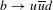transitions, as shown in Fig. 1. Throughout this paper the inclusion of charge-conjugate reactions is implied, unless otherwise indicated. CPV could arise from the interference of two amplitudes with relative phases that differ between particle and antiparticle decays, leading to differences in the Λb0 and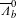decay rates. The main source of this effect in the SM would be the large relative phase (referred to as α in the literature) between the product of the CKM matrix elements VubVud and VtbVtd, which are present in the different diagrams depicted in Fig. 1. Parity violation (PV) is also expected in weak interactions, but has never been observed in Λb0 decays.Figure 1: Dominant Feynman diagrams for Λb0 → pπ−π+π− and Λb0 → pπ−K+K− transitions.

To search for CP-violating effects one needs to measure CP-odd observables, which can be done by studying asymmetries in the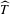operator. This is a unitary operator that reverses both the momentum and spin three-vectors17,18, and is different from the antiunitary time-reversal operator T19,20 that also exchanges initial and final states. A non-zero CP-odd observable implies CP violation, and similar considerations apply to P-odd observables and parity violation21. Furthermore, different values of P-odd observables for a decay and its charge conjugate would imply CPV. In this paper, scalar triple products of final-state particle momenta in the Λb0 centre-of-mass frame are studied to search for P- and CP-violating effects in four-body decays. These are defined as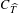= pp (ph1 × ph2+) for Λb0 and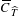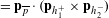for, where h1 and h2 are final-state hadrons: h1 = π and h2 = K for Λb0K+K and h1 = h2 = π for Λb0π+π. In the latter case there is an inherent ambiguity in the choice of the pion for h1 that is resolved by taking that with the larger momentum in the Λb0 rest frame, referred to as πfast. The following asymmetries may then be defined22,23: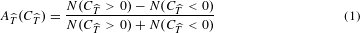where N and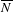are the numbers of Λb0 anddecays. These asymmetries are P-odd and-odd and so change sign under P ortransformations, that is,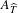()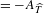(−) or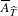() = −(−). The P- and CP-violating observables are defined as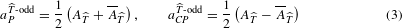and a significant deviation from zero would signal PV or CPV, respectively.

Searches for CPV with triple-product asymmetries are particularly suited to Λb0 four-body decays to hadrons with no charm quark24 thanks to the rich resonant substructure, dominated by Δ(1232)+++ and ρ(770)0π+π resonances in the Λb0π+π final state. The observable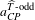is sensitive to the interference of-even and-odd amplitudes with different CP-odd (‘weak’) phases. Unlike the overall asymmetry in the decay rate that is sensitive to the interference of-even amplitudes,does not require a non-vanishing difference in the CP-invariant (‘strong’) phase between the contributing amplitudes19,25. The observables,,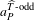andare, by construction, largely insensitive to particle–antiparticle production asymmetries and detector-induced charge asymmetries26.

This article describes measurements of the CP- and P-violating asymmetries introduced in equation (3) in Λb0π+π and Λb0K+K decays. The asymmetries are measured first for the entire phase space of the decay, integrating over all possible final-state configurations, and then in different regions of phase space so as to enhance sensitivity to localized CPV. The analysis is performed using proton–proton collision data collected by the LHCb detector, corresponding to 3.0 fb−1 of integrated luminosity at centre-of-mass energies of 7 and 8 TeV, and exploits the copious production of Λb0 baryons at the LHC, which constitutes around 20% of all b hadrons produced27. Control samples of Λb0pKπ+π and Λb0Λc+π decays, with Λc+ decaying to pKπ+, π+, and pKK+ final states, are used to optimize the event selection and study systematic effects; the most abundant control sample consists of Λb0Λc+(pKπ+)π decays mediated by bc quark transitions in which no CPV is expected28. To avoid introducing biases in the results, all aspects of the analysis, including the selection, phase space regions, and procedure used to determine the statistical significance of the results, were fixed before the data were examined.

The LHCb detector29,30 is designed to collect data of b-hadron decays produced from proton–proton collisions at the Large Hadron Collider. It instruments a region around the proton beam axis, covering the polar angles between 10 and 250 mrad, where approximately 24% of the b-hadron decays occur31. The detector includes a high-precision tracking system with a dipole magnet, providing measurements of the momentum and decay vertex position of particle decays. Different types of charged particles are distinguished using information from two ring-imaging Cherenkov detectors, a calorimeter and a muon system. Simulated samples of Λb0 signal modes and control samples are used in this analysis to verify the experimental method and to study certain systematic effects. These simulated events model the experimental conditions in detail, including the proton–proton collision, the decays of the particles, and the response of the detector. The software used is described in refs 32,33,34,35,36,37,38. The online event selection is performed by a trigger system that takes fast decisions about which events to record. It consists of a hardware stage, based on information from the calorimeter and muon systems, followed by a software stage, which applies a full event reconstruction. The software trigger requires Λb0 candidates to be consistent with a b-hadron decay topology, with tracks originating from a secondary vertex detached from the primary pp collision point. The mean Λb0 lifetime is 1.5 ps (ref. 39), which corresponds to a typical flight distance of a few millimetres in the LHCb.

The Λb0h+h candidates are formed by combining tracks identified as protons, pions, or kaons that originate from a common vertex. The proton or antiproton identifies the candidate as a Λb0 or. There are backgrounds from b-hadron decays to charm hadrons that are suppressed by reconstructing the appropriate two- or three-body invariant masses, and requiring them to differ from the known charm hadron masses by at least three times the experimental resolution. For the Λb0Λc+π control mode, only the Λb0ph+hπ events with reconstructed ph+h invariant mass between 2.23 and 2.31 GeV/c2are retained.

A boosted decision tree (BDT) classifier40 is constructed from a set of kinematic variables that discriminate between signal and background. The signal and background training samples used for the BDT are derived from the Λb0pKπ+π control sample, since its kinematics and topology are similar to the decays under study; background in this sample is subtracted with the sPlot technique41, a statistical technique to disentangle signal and background contributions. The background training sample consists of candidates that lie far from the signal mass peak, between 5.85 and 6.40 GeV/c2. The control modes Λb0Λc+(+π)π and Λb0Λc+(pKK+)π are used to optimize the particle identification criteria for the signal mode with the same final state. For events in which multiple candidates pass all selection criteria for a given mode, one candidate is retained at random and the rest discarded.

Unbinned extended maximum likelihood fits to the π+π and the K+K invariant mass distributions are shown in Fig. 2. The invariant mass distribution of the Λb0 signal is modelled by a Gaussian core with power-law tails42, with the mean and the width of the Gaussian determined from the fit to data. The combinatorial background is modelled by an exponential distribution with the rate parameter extracted from data. All other parameters of the fit model are taken from simulations except the yields. Partially reconstructed Λb0 decays are described by an empirical function43 convolved with a Gaussian function to account for resolution effects. The shapes of backgrounds from other b-hadron decays due to incorrectly identified particles, for example, kaons identified as pions or protons identified as kaons, are modelled using simulated events. These consist mainly of Λb0pKπ+π and B0K+πππ+ decays for the Λb0π+π sample and of similar final states for the Λb0K+K sample, as shown in Fig. 2. The yields of these contributions are obtained from fits to data reconstructed under the appropriate mass hypotheses for the final-state particles. The signal yields of Λb0π+π and Λb0K+K are 6,646 ± 105 and 1,030 ± 56, respectively. This is the first observation of these decay modes.Figure 2: Reconstructed invariant mass fits used to extract the signal yields.

Signal candidates are split into four categories according to Λb0 orflavour and the sign oforto calculate the asymmetries defined in equations (1) and (2). The reconstruction efficiency for signal candidates with> 0 is identical to that with< 0 within the statistical uncertainties of the control sample, and likewise for, which indicates that the detector and the reconstruction program do not bias this measurement. This check is performed both on the Λb0Λc+(pKπ+)π data control sample and on large samples of simulated events, using yields about 30 times those found in data, which are generated with no CP asymmetry. The CP asymmetry measured in the control sample is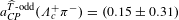%, compatible with CP symmetry. The asymmetriesandin the signal samples are measured with a simultaneous unbinned maximum likelihood fit to the invariant mass distributions of the different signal categories, and are found to be uncorrelated. Corresponding asymmetries for each of the background components are also measured in the fit; they are found to be consistent with zero, and do not lead to significant systematic uncertainties in the signal asymmetries. The values ofandare then calculated fromand.

In four-body particle decays, the CP asymmetries may vary over the phase space due to resonant contributions or their interference effects, possibly cancelling when integrated over the whole phase space. Therefore, the asymmetries are measured in different regions of phase space for the Λb0π+π decay using two binning schemes, defined before examining the data. Scheme A, defined in Table 1, is designed to isolate regions of phase space according to their dominant resonant contributions. Scheme B exploits in more detail the interference of contributions which could be visible as a function of the angle Φ between the decay planes formed by the fast and the πslowπ+ systems, as illustrated in Fig. 3. Scheme B has ten non-overlapping bins of width π/10 in | Φ | . For every bin in each of the schemes, the Λb0 efficiencies for> 0 and< 0 are compared and found to be equal within uncertainties, and likewise theefficiencies for> 0 and< 0. The analysis technique is validated on the Λb0Λc+(pKπ+)π control sample, for which the angle Φ is defined by the decay planes of the pK and π+π pairs, and on simulated signal events.

The asymmetries measured in Λb0π+π decays with these two binning schemes are shown in Fig. 4 and reported in Table 2, together with the integrated measurements. For each scheme individually, the compatibility with the CP-symmetry hypothesis is evaluated by means of a χ2 test, with χ2 = RTV−1R, where R is the array ofmeasurements and V is the covariance matrix, which is the sum of the statistical and systematic covariance matrices. An average systematic uncertainty, whose evaluation is discussed below, is assigned for all bins. The systematic uncertainties are assumed to be fully correlated; their contribution is small compared to the statistical uncertainties. The p-values of the CP-symmetry hypothesis are 4.9 × 10−2 and 7.1 × 10−4 for schemes A and B, respectively, corresponding to statistical significances of 2.0 and 3.4 Gaussian standard deviations (σ). A similar χ2 test is performed onmeasurements with p-values for the P-symmetry hypothesis of 5.8 × 10−3 (2.8σ) and 2.4 × 10−2 (2.3σ), for scheme A and B, respectively. The overall significance for CPV in Λb0π+π decays from the results of schemes A and B is determined by means of a permutation test44, taking into account correlations among the results. A sample of 40,000 pseudoexperiments is generated from the data by assigning each event a random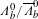flavour such that CP symmetry is enforced. The sign ofis unchanged if a Λb0 candidate stays Λb0 and reversed if the Λb0 candidate becomes. The p-value of the CP-symmetry hypothesis is determined as the fraction of pseudoexperiments with χ2 larger than that measured in data. Applying this method to the χ2 values from schemes A and B individually, the p-values obtained agree with those from the χ2 test within the uncertainty due to the limited number of pseudoexperiments. To assess a combined significance from the two schemes, the product of the two p-values measured in data is compared with the distribution of the product of the p-values of the two binning schemes from the pseudoexperiments. The fraction of pseudoexperiments whose p-value product is smaller than that seen in data determines the overall p-value of the combination of the two schemes45. An overall p-value of 9.8 × 10−4 (3.3σ) is obtained for the CP-symmetry hypothesis, including systematic uncertainties.

The main sources of systematic uncertainties for both π+π and K+K decays are experimental effects that could introduce biases in the measured asymmetries. This is tested by measuring the asymmetry, integrated over phase space and in various phase space regions, using the control sample Λb0Λc+(pKπ+)π, which is expected to exhibit negligible CPV. The results are in agreement with the CP-symmetry hypothesis; an uncertainty of 0.31% is assigned as a systematic uncertainty for theandintegrated measurements; an uncertainty of 0.60%, the largest asymmetry from a fit to scheme B measurements using a range of efficiency and fit models, is assigned for the corresponding phase space measurements. The systematic uncertainty arising from the experimental resolution in the measurement of the triple productsand, which could introduce a migration of events between the bins, is estimated from simulated samples of Λb0π+π and Λb0K+K decays where neither P- nor CP-violating effects are present. The difference between the reconstructed and generated asymmetry is taken as a systematic uncertainty due to this effect, and is less than 0.06% in all cases. To assess the uncertainty associated with the fit models, alternative functions are used; these tests lead only to small changes in the asymmetries, the largest being 0.05%. For Λb0K+K decays, this contribution is larger, about 0.28% for theandasymmetries.